# Array Interfaces in Julia

• Last Updated : 12 Oct, 2020

An array interface is a syntactical contract for arrays in Julia that they must follow. This article describes the various methods which can be adopted to construct array interfaces in Julia. It also explains how to perform indexing in an array interface to access its elements.

An array interface can be constructed by taking a standard array and linking custom keys along with the indices of each dimension. This can be achieved by using the AxisIndices package.

Construction of an Array Using AxisArray Function:

An array can be created using the AxisArray() function present in the AxisIndices package of Julia.

Example:

## Julia

 `# Julia program to declare an array interface  `` ` `# Importing AxisIndices package``using AxisIndices`` ` `# Declare array``arr ``=` `[``5` `6``; ``7` `8``];`` ` `# Using AxisArray function to return an array ``# with its dimension names and axis values``axis ``=` `AxisArray(arr, [``"row1"``, ``"row2"``], ``                      ``[:col1, :col2])`

Output: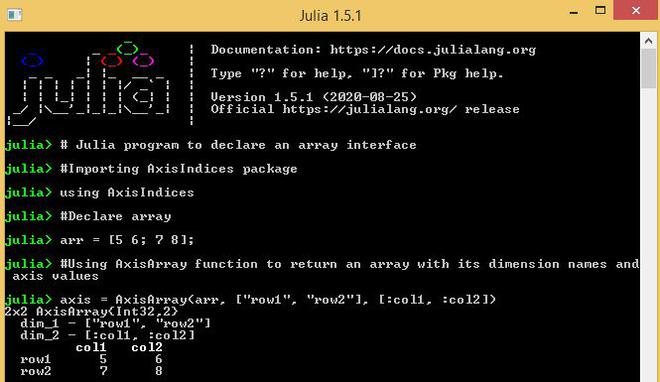An array interface can also be initialized using the following syntax:

Syntax:

```Array{T}(undef, dims)
```

Example:

## Julia

 `# Julia program to declare an array interface  `` ` `# Importing AxisIndices package``using AxisIndices`` ` `# Declare array``arr ``=` `[``5` `6``; ``7` `8``];`` ` `# Using AxisArray function to return an array ``# with its dimension names and axis values``axis ``=` `AxisArray{``Int``}(undef, [``"row1"``, ``"row2"``], ``                             ``[:col1, :col2]);`` ` `axis[:, :] ``=` `arr;`` ` `axis`

Output: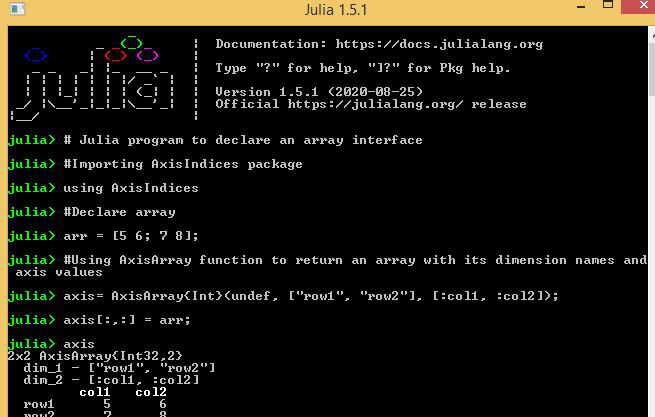Construction of an Array Using NamedAxisArray() Function:

Each Dimension or axis of the array interface can be given names using NamedAxisArray present in the AxisIndices package of Julia.

Example:

## Julia

 `# Julia program to declare an array interface  `` ` `# Importing AxisIndices package``using AxisIndices`` ` `# Declare array``arr ``=` `[``5` `6``; ``7` `8``];`` ` `# Using AxisArray function to return an array ``# with its dimension names and axis values``axis ``=` `AxisArray(arr, [``"row1"``, ``"row2"``], ``                      ``[:col1, :col2])`` ` `# Using NamedAxisArray function``# to return an array with named axes``axis_named ``=` `NamedAxisArray{(:xdim, :ydim)}(axis)`

Output: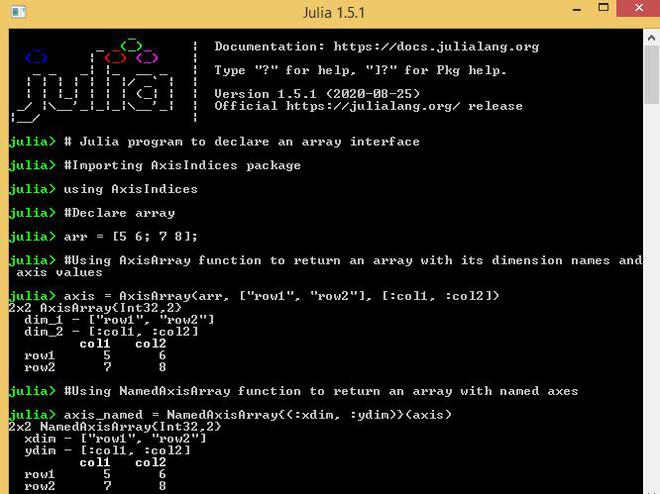Construction of an Array Using MetaAxisArray() Function:

Example:

## Julia

 `# Julia program to declare an array interface  `` ` `# Importing AxisIndices package``using AxisIndices`` ` `# Declare array``arr ``=` `[``5` `6``; ``7` `8``];`` ` `# Using MetaAxisArray function to return ``# an array with its dimension names, ``# axis values, metadata``axis_meta ``=` `MetaAxisArray(arr, ([``"row1"``, ``"row2"``], ``                                ``[:col1, :col2]), ``                          ``metadata ``=` `"Array Interface"``)`

Output: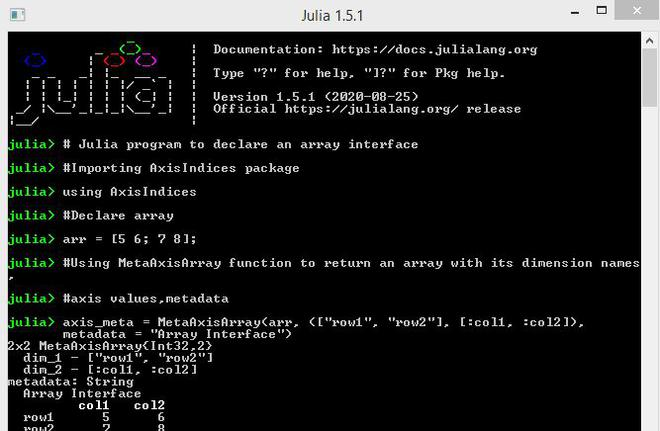#### Indexing of Arrays

Indexing in an Array Interface Using Unitful Package:

An array interface can be indexed just like standard arrays in Julia. This can be achieved by using the Unitful package.

Example:

## Julia

 `# Julia program to perform indexing ``# in an array interface`` ` `# Importing AxisIndices package``using AxisIndices`` ` `# Importing Unitful package``import` `Unitful: m`` ` `# Using reshape function ``# to return an array with ``# its specified dimensions``arr ``=` `reshape(``1``:``6``, ``2``, ``3``);`` ` `# Using AxisArray function to return ``# an array with its dimension names ``# and axis values``axis ``=` `AxisArray(arr, ((``1``:``2``)m,``                ``[``"col1"``, ``"col2"``, ``"col3"``]))`

Output: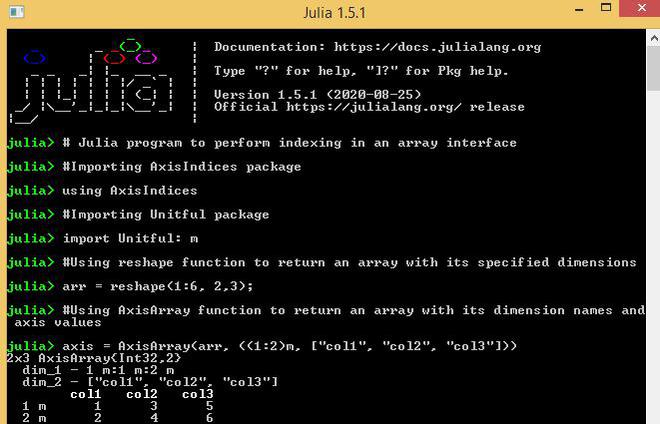Example:

## Julia

 `# Julia program to perform indexing ``# in an array interface `` ` `# Importing AxisIndices package``using AxisIndices`` ` `# Importing Unitful package``import` `Unitful: m`` ` `# Using reshape function``# to return an array``# with its specified dimensions``arr ``=` `reshape(``1``:``6``, ``2``, ``3``);`` ` `# Using AxisArray function``# to return an array ``# with its dimension names and axis values``axis ``=` `AxisArray(arr, ((``1``:``2``)m, ``                ``[``"col1"``, ``"col2"``, ``"col3"``]))`` ` `# displaying only the first row ``# and all the columns``axis[``1``,:]`

Output: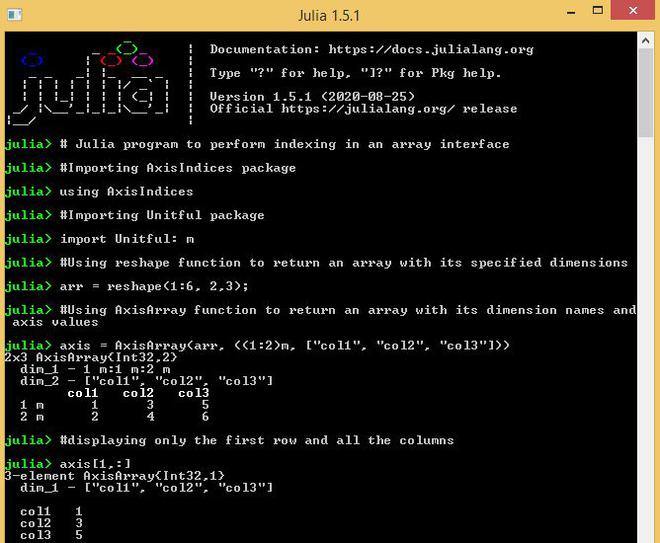Example:

## Julia

 `# Julia program to perform indexing ``# in an array interface `` ` `# Importing AxisIndices package``using AxisIndices`` ` `# Importing Unitful package``import` `Unitful: m`` ` `# Using reshape function to return ``# an array with its specified dimensions``arr ``=` `reshape(``1``:``6``, ``2``, ``3``);`` ` `# Using AxisArray function to return``# an array with its dimension names ``# and axis values``axis ``=` `AxisArray(arr, ((``1``:``2``)m, ``                ``[``"col1"``, ``"col2"``, ``"col3"``]))`` ` `# displaying the first two rows``# and the first two columns``axis[``1``:``2``, ``1``:``2``]`

Output: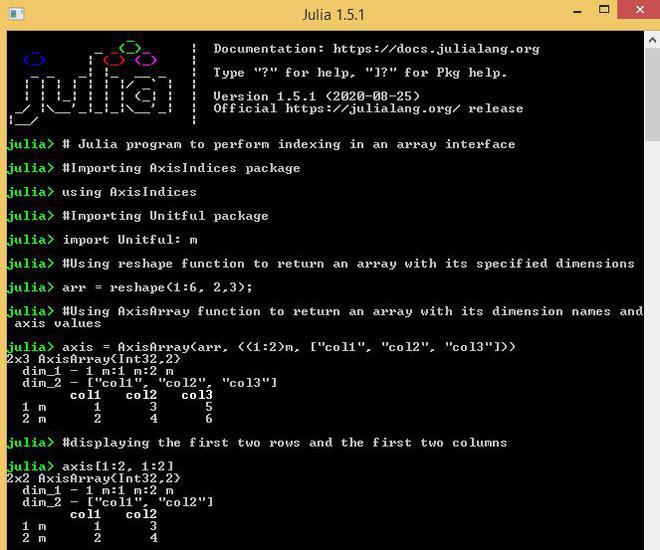Example:

## Julia

 `# Julia program to perform indexing``# in an array interface`` ` `# Importing AxisIndices package``using AxisIndices`` ` `# Importing Unitful package``import` `Unitful: m`` ` `# Using reshape function to return ``# an array with its specified dimensions``arr ``=` `reshape(``1``:``6``, ``2``, ``3``);`` ` `# Using AxisArray function to return``# an array with its dimension names and axis values``axis ``=` `AxisArray(arr, ((``1``:``2``)m, ``                ``[``"col1"``, ``"col2"``, ``"col3"``]))`` ` `# displaying the first 3 elements of the array``axis[``1``:``3``]`

Output: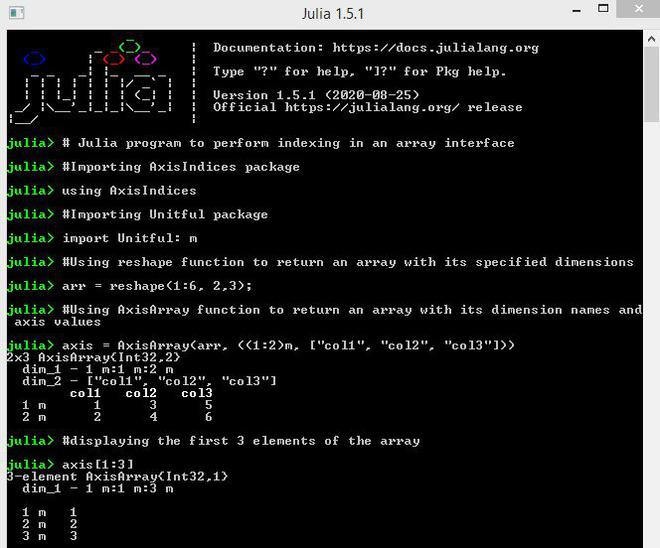Indexing in an Array Interface Using Keys:

An Array Interface can be indexed with the help of keys.

Example :

## Julia

 `# Julia program to perform indexing ``# in an array interface`` ` `# Importing AxisIndices package``using AxisIndices`` ` `# Importing Unitful package``import` `Unitful: m`` ` `# Using reshape function to return``# an array with its specified dimensions``arr ``=` `reshape(``1``:``6``, ``2``, ``3``);`` ` `# Using AxisArray function to return``# an array with its dimension names and axis values``axis ``=` `AxisArray(arr, ((``1``:``2``)m, ``                ``[``"col1"``, ``"col2"``, ``"col3"``]))`` ` `# accessing an element using its specified keys``axis[``1m``, ``"col1"``]`

Output: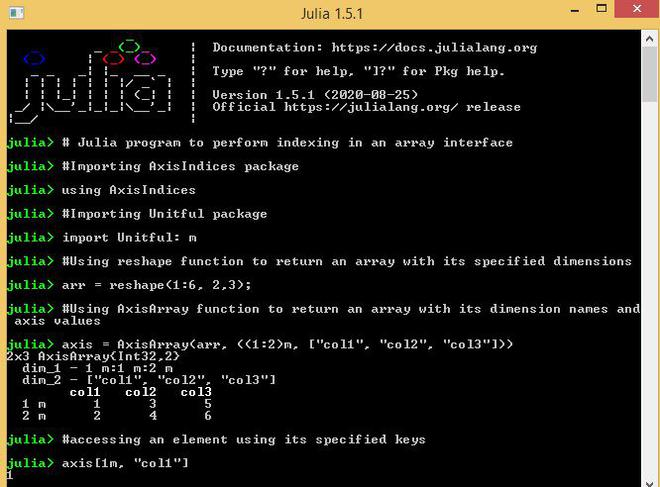Indexing in an Array Interface Using Filtration Functions:

An array interface can also be indexed using functions that filter the keys.

Example:

## Julia

 `# Julia program to perform indexing``# in an array interface`` ` `# Importing AxisIndices package``using AxisIndices`` ` `# Importing Unitful package``import` `Unitful: m`` ` `# Using reshape function to return ``# an array with its specified dimensions``arr ``=` `reshape(``1``:``6``, ``2``, ``3``);`` ` `# Using AxisArray function to return ``# an array with its dimension names and axis values``axis ``=` `AxisArray(arr, ((``1``:``2``)m, ``                ``[``"col1"``, ``"col2"``, ``"col3"``]))`` ` `# Applying filters to the keys``axis[!``=``(``1m``), ``in``([``"col1"``, ``"col2"``])]`

Output: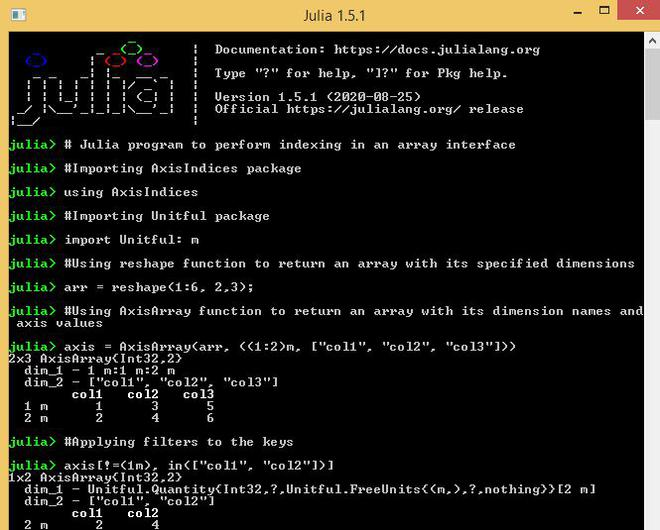My Personal Notes arrow_drop_up